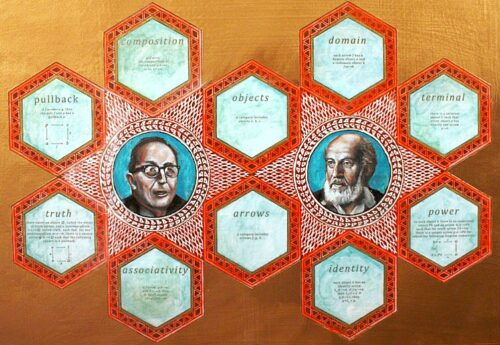### BIJIJOO/ Paintings/ The Chamber of Scientists / Category TheoryIn 1942–45, mathematicians Samuel Eilenberg (right) and Saunders Mac Lane (left), introduced category theory as part of their work in algebraic topology. Category theory abstractly codifies the properties of mathematical concepts by formalising them as collections of objects and arrows (morphisms) which satisfy certain basic conditions. Significant areas of mathematics can be formalised as categories, and the use of category theory allows many intricate and subtle mathematical results in these fields to be stated, and proved, in a much simpler and elegant way than without the use of categories.

Categories can be used as a possible foundation of all of mathematics, thus replacing the use in such a foundation of the usual Zermelo-Fraenkel axioms for set theory. The category theory axioms orbit the heads of Eilenberg and Mac Lane shown in the painting.